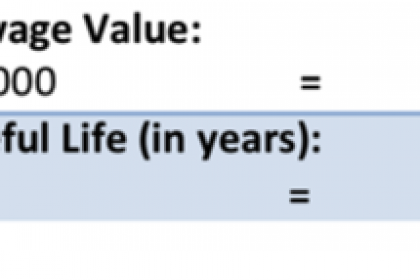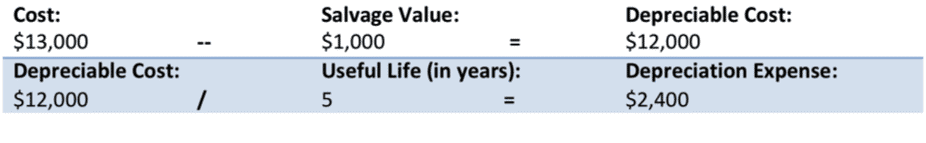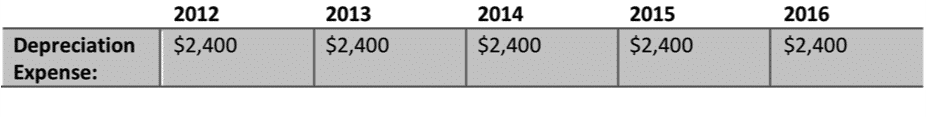# Depreciation- Small Business Accounting Tutorial## Depreciation: Overview

Most companies own assets that have long useful lives. These can take the form of buildings, equipment, and vehicles. As explained in previous lessons, companies must follow the cost principle of GAAP (recording assets at cost). In order to allocate the cost of using the asset over a length of time, companies use depreciation. Depreciation is the process of allocating the cost of an asset to expense over its useful life. However, in terms of accounting, note that this does not show the actual value of the asset. This is simply a way to allocate its cost.

There are multiple methods to account for depreciation. The most common method is straight-line. Under the straight-line method, companies expense an equal amount of depreciation each year of the asset’s useful life. While this amount may not match the asset’s actual value, it is a good method for accounting for the expense. Let us look at an example.

Company XYZ Inc purchased a delivery truck for \$13,000. In order to compute the annual depreciation expense, we divide the depreciable cost by the estimated useful life. Based on the condition of the truck, XYZ Inc estimates that its useful life is 5 years. So XYZ Inc takes the cost of the truck and subtracts its salvage value (let’s say \$1,000) to find the depreciable cost: \$12,000. Then, XYZ takes the truck’s depreciable cost (\$12,000) and divides it by its useful life in years (5) to find its annual depreciation expense: \$2,400.###### Graphic #1: Calculating straight-line amounts for an asset.

If XYZ’s accounting period ends on December 31, the company will report the depreciation expense on the company’s income statement as shown in the following depreciation schedule:###### Graphic #2: Creating a schedule for an asset.

There are also other methods of depreciation. The declining-balance method uses an accelerated-depreciation method because it results in high depreciation in the early years of an asset’s life. The units-of-activity method takes into consideration the units of production from a specific asset. These methods are much more complex and may require help from an accountant.

## Depreciation: Video Lesson

The following video lesson, titled “Depreciation,” is the corresponding video lesson to the material shown above in the “Overview” section. This video is from our complete accounting tutorial, titled “Mastering Accounting Made Easy v.2.0.”

TOP
Sale! \$49 All-Access 1 Days 10 Hours 53 Minutes 14 Seconds      \$199 \$49 Entire Library!